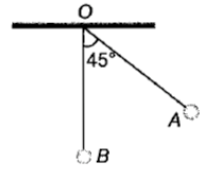The bob A of a simple pendulum is released when the string makes an angle of 450 with the vertical. It hits another bob B of the same material and the same mass kept at rest on a table. If the collision is elastic1.  Both A and B rise to the same height

2.  Both A and B come to rest at B

3.  Both A and B move with the same velocity of A

4.  A comes to rest and B moves with the velocity of A

Concept Questions :-

Linear momentum
High Yielding Test Series + Question Bank - NEET 2020

Difficulty Level:

If a force acts on a body at a point away from the centre of mass, then

1.  Linear acceleration changes

2.  Angular acceleration changes

3.  Both change

4.  None of these

Concept Questions :-

Torque
High Yielding Test Series + Question Bank - NEET 2020

Difficulty Level:

If the linear density of a rod of length 3m varies as $\lambda$=2+x, then the position of the center of mass of the rod is at a distance of

1.  $\frac{7}{3}m$

2.  $\frac{10}{7}m$

3.  $\frac{12}{7}m$

4.  $\frac{9}{7}m$

Concept Questions :-

Center of mass
High Yielding Test Series + Question Bank - NEET 2020

Difficulty Level:

A sphere cannot roll on

1.  a smooth horizontal surface

2.  a rough horizontal surface

3.  a smooth inclined surface

4.  a rough inclined surface

Concept Questions :-

Rolling motion
High Yielding Test Series + Question Bank - NEET 2020

Difficulty Level:

Two balls are thrown simultaneously in the air. The acceleration of the center of mass of the two balls while in air

1.  depends on the direction of the motion of the balls

2.  depends on the masses of the two balls

3.  depends on the speeds of two balls

4.  is equal to g

Concept Questions :-

Center of mass
High Yielding Test Series + Question Bank - NEET 2020

Difficulty Level:

A cord is wound round the circumference of wheel of radius r. The axis of the wheel is horizotal and moment of inertia about it is I. A weight mg is attached to the end of the cord and falls from rest. After falling through a distance h, the angular velocity of the wheel will be

(1) $\sqrt{\frac{2gh}{I+mr}}$

(2) $\sqrt{\frac{2mgh}{I+m{r}^{2}}}$

(3) ${\left[\frac{2mgh}{I+2m}\right]}^{\frac{1}{2}}$

(4) $\sqrt{2gh}$

Concept Questions :-

Rolling motion
High Yielding Test Series + Question Bank - NEET 2020

Difficulty Level:

If a body of mass m collides head on, elastically with velocity u with another identical body at rest. After collision velocity of the second body will be

(1) zero

(2) u

(3) 2u

(4) data insufficient

Concept Questions :-

Center of mass
High Yielding Test Series + Question Bank - NEET 2020

Difficulty Level:

A particle of mass m at rest is acted upon by a constant force F for a time t. Its kinetic energy after an interval t is

1.  $\frac{{\mathrm{F}}^{2}{\mathrm{t}}^{2}}{\mathrm{m}}$

2.  $\frac{{\mathrm{F}}^{2}{\mathrm{t}}^{2}}{2\mathrm{m}}$

3.  $\frac{{\mathrm{F}}^{2}{\mathrm{t}}^{2}}{3\mathrm{m}}$

4.  $\frac{\mathrm{Ft}}{2\mathrm{m}}$

Concept Questions :-

Linear momentum
High Yielding Test Series + Question Bank - NEET 2020

Difficulty Level:

If a person standing on a rotating disc stretches out his hands, the angular speed will

1.  increase

2.  decrease

3.  remain same

4.  None of these

Concept Questions :-

Angular momentum
High Yielding Test Series + Question Bank - NEET 2020

Difficulty Level:

If the earth were to suddenly contract to $\frac{1}{n}$th of its present size without any change in its mass, the duration of the new day will be nearly

1.  24/n hours

2.  24 n hours

3.  24/${\mathrm{n}}^{2}$ hours

4.   24 ${\mathrm{n}}^{2}$ hours

Concept Questions :-

Angular momentum